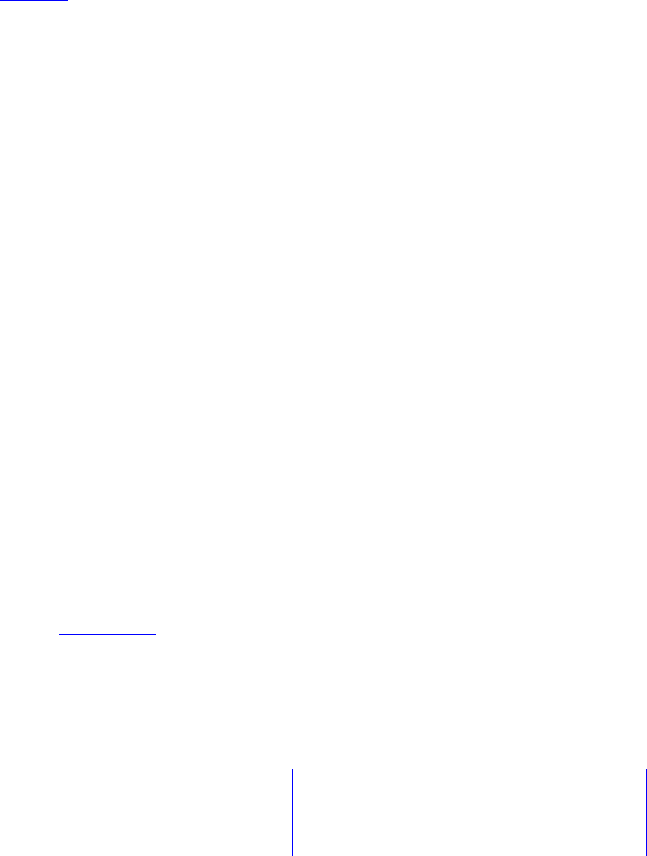Study Guides (400,000)
CA (150,000)
U of S (700)
MATH (30)
Scott (8)
Final

# MATH 125 Study Guide - Final Guide: Row Echelon Form, Invertible Matrix, Elementary Matrix

Department
Mathematics
Course Code
MATH 125
Professor
Scott
Study Guide
Final

This preview shows pages 1-3. to view the full 36 pages of the document.MATH 125 (R1) Winter 2017
Additional Practice Problems for Final – Solutions
Notes: Some of the solutions here are incomplete (their main purpose is to enable you
to check your own answers). In particular, row reduction of matrices and determinant
calculations are omitted for the most part. In your exam, however, you should show all your
work, and give suitable justiﬁcation for your answers. Note also that there are often multiple
ways to solve a particular problem; in these solutions, we typically just give one approach.
1. a) Deﬁne what it means for an n×nmatrix to be invertible.
b) Find the inverse (if it exists) of the matrix
A=
1 0 1
0 1 0
2 1 1
c) For which value(s) of ais the matrix
1 0 a
0 1 a
2 1 a
Solution: (a) An n×nmatrix Ais invertible if there exists another n×nmatrix B
such that AB =In=BA, where Inis the n×nidentity matrix.
(b) We use the standard algorithm:
1 0 1 1 0 0
0 1 0 0 1 0
2 1 1 0 0 1
→ · · · −
1 0 0 11 1
0 1 0 010
0 0 1 2 1 1
Since the reduced row echelon form of Ais I3(the left side of the partitioned matrix),
Ais invertible and
A1=
11 1
010
2 1 1
(the right side of the partitioned matrix).
(c) Let Bbe the given matrix. Then:

Only pages 1-3 are available for preview. Some parts have been intentionally blurred.det(B) =
1 0 a
0 1 a
0 1 a
(r32r1)
=
1a
1a
(expand along 1st column)
=2a.
Hence Bis invertible if and only if 2a6= 0, i.e., if and only if a6= 0.
2. Let A=
13 6
25 11
1 1 3
.
a) Ais invertible. Compute A1.
b) Use A1to solve the following system of equations:
x13x2+ 6x3= 1
2x15x2+ 11x3=3
x1+x23x3= 1
Solution: (a) We use the standard algorithm:
13 6 1 0 0
25 11 0 1 0
1 1 3 0 0 1
→ · · · −
1 0 0 4 33
0 1 0 531
0 0 1 321
Since the reduced row echelon form of Ais I3(the left side of the partitioned matrix),
Ais invertible and
A1=
433
531
321
(the right side of the partitioned matrix).
(b) The matrix equation of the system is A~x =
1
3
1
, so by (a), the unique solution
of the system is
~x =A1
1
3
1
=
433
531
321
1
3
1
=
10
13
8
.

Only pages 1-3 are available for preview. Some parts have been intentionally blurred.3. Compute the determinant of each of the following matrices:
a)
3 7 0 6
7 9 0 3
93 0 5
1 5 0 3
b)
123
123
414
c)
2 0 0 0
1 6 0 0
5 9 1 0
7 8 3 3
d)
1 0 1 0
0 1 0 1
1 1 1 2
1 1 0 1
e)
12304
01001
1 1 1 1 2
12 1 0 1
00102
Solution: (a) Since the matrix contains a column of zeros, its determinant is 0.
(b) Since the matrix contains a row which is a multiple of the other (r2=r1), its
determinant is 0.
(c) Since the matrix is triangular, its determinant is the product of its diagonal entries,
i.e., (2)(6)(1)(3) = 36.
(d) The determinant is 1 (standard calculation omitted: use cofactor expansion/row
operations/column operations or a combination of all these methods).
(e) The determinant is 5 (standard calculation omitted: use cofactor expansion/row
operations/column operations or a combination of all these methods).
4. Find the rank and nullity of
1 6 11 16 21
3 8 13 18 23
5 10 15 20 25
7 12 17 22 27
9 14 19 24 29
(Hint: The rank is 2, and it shouldn’t take many computations to see this). What is
the determinant of the matrix?
Solution: Denote the given matrix by A. Observe that each row of Ais obtained from
the previous one by adding 2 to each entry. Thus: